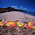Thursday, December 13, 2012

Introduction:

Apriori is a very basic and straight forward algorithm for frequent pattern mining, I will not be discussing much about the approach, as those can already be studied from different lectures/books available on net. I will basically present an implementation of mine which is an efficient implementation of the standard apriori algorithm in Java. The target input files are taken from Frequent Itemset Mining Dataset Repository, for example, the mushroom dataset. Due to the space and runtime complexity, apriori is not suitable for larger files having 50,000 records or so. It also may take huge time for very dense files.

The Database:

First we need to read the files and store them in some data structure which will be easy to access and efficient at the same time. Here. I am presenting my java source code, and then I will present an explaining analysis why this works better.
```/*
Author: Zobayer Hasan
*/

import java.io.FileInputStream;
import java.util.ArrayList;
import java.util.Collections;
import java.util.Comparator;
import java.util.List;
import java.util.PriorityQueue;

class Entry {
public Integer first;
public Integer second;
Entry() {}
Entry(Integer first, Integer second) {
this.first = first;
this.second = second;
}
}

public class Database {
public static boolean debugger = false;

private final List< List< Integer > > transactions;
private final List< Integer > items;

public Database(String dataFileName) throws Exception {
if(debugger) {
System.out.println("Processing " + dataFileName);
}

transactions = new ArrayList< List< Integer > >();
items = new ArrayList< Integer >();

FileInputStream fin = new FileInputStream(dataFileName);

String line;

double startTime = System.currentTimeMillis();

while((line = stdin.readLine()) != null) {
List< Integer > transaction = new ArrayList< Integer >();
String[] temp = line.split("\\s+");

for(String num : temp) {
}

if(transaction.isEmpty()) continue;

Collections.sort(transaction);
}

fin.close();
istream.close();
stdin.close();

int n = transactions.size();
PriorityQueue< Entry > pQ = new PriorityQueue< Entry >(n, new Comparator< Entry >() {
public int compare(Entry item1, Entry item2) {
if(item1.first.equals(item2.first)) {
return item1.second.compareTo(item2.second);
} else {
return item1.first.compareTo(item2.first);
}
}
});

for(int i = 0; i < n; i++) {
}

while(!pQ.isEmpty()) {
Entry peek = pQ.remove();
int val = peek.first;
int idx = peek.second;
if(items.isEmpty() || items.get(items.size()-1) < val) {
}
}
}
}

double endTime = System.currentTimeMillis();
System.out.println("Database created in " + (endTime - startTime)/1000.0 + " seconds");
}

public int scanDatabase(List< Integer > transaction) {
int count = 0;
for(List< Integer > row : transactions) {
boolean found = true;
for(Integer item : transaction) {
int idx, stp, st = 0, en = row.size(), cnt = en - st;
while(cnt > 0) {
stp = cnt >> 1; idx = st + stp;
if(row.get(idx).compareTo(item) < 0) {
st = ++idx;
cnt -= stp+1;
}
else {
cnt = stp;
}
}
if(st == row.size() || row.get(st).compareTo(item) != 0) {
found = false;
break;
}
}
if(found) count++;
}
return count;
}

public List< Integer > getItemset() {
return items;
}

public int dbSize() {
return transactions.size();
}

public List< Integer > getRow(int row) {
try {
return transactions.get(row);
} catch(Exception e) {
throw e;
}
}
}
```
Clearly it provides some interface to access the database where the constructor must be called with a file name for example 'mushroom.dat'. The sorting on line 56 is not necessary if you know the transactions in the file will be sorted in ascending order. All the data files in this repository are already sorted, so sorting can be disabled.

Now, if we look at the constructor, what is this huge code doing actually? First it treads each transaction as a string, then parses it and inserts the transaction in a sorted list. Now, we need a list of unique elements. Well, this could be done by sorting all the records together, and then eliminating duplicates. However, this can be performed better with the help of a priority queue in O(NK log N) time, where we have n transactions, and each transaction has K records on an average. Actually this works much better than it would be in naive approach which would take O(NK log NK) because of extra sorting overhead.

Then comes the last important part, scanDatabase() method. Which basically searches each record in the database for a set of elements. It takes O(NK log K) time at most, where, we have N records, each having an average length of K. This is much better than looking in O(N*K^2) following a naive approach. Here is some improvement possible, if we know the length of transactions, we could use a bit vector in addition to each transaction. Then the query can be performed in O(NK). However, as the datasets are huge, I didn't go for it due to the necessity of using huge memory.

Part 2 contains the source code and explanation for the apriori algorithm.

1.can apriori algorithm be used for text file mining

2.Algorithms works on data, text files have data.How hard is Finding a way?

3.can i get the code for mining text data?

4.Dude do u have the code for determining transitional patterns

5.can anyone mail me javacode for FIN,FPGROWTH AND PREPOST algorithm please send me if anybody knows because i have to complete my project